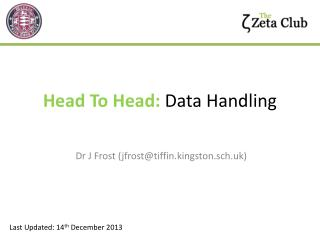DownloadDownload PresentationTélécharger la présentation- - - - - - - - - - - - - - - - - - - - - - - - - - - E N D - - - - - - - - - - - - - - - - - - - - - - - - - - -
##### Presentation Transcript

1. Head To Head: Data Handling Dr J Frost (jfrost@tiffin.kingston.sch.uk) Last Updated: 14th December 2013

2. Head to Head You are head to head with the person on your table. You must circle or underline your answer to finalise it. Once you finalise an answer, you can’t change it. The first person with the correct answer wins. If both people get it wrong, just do a quick paper-scissors-rock to settle. If neither person answers, the person who previously won wins. Winner moves up (as per left). Loser moves down. vs Head Table 8 9 Rear Table 2 7 10 15 3 6 11 14 4 5 12 13

3. Question 1 What is the lower quartile of the following numbers? 1 2 3 4 5 6 7 8 9 ? Answer: 2.5 (Click above to reveal answer)

4. Question 2 What percentage of data is above the upper quartile? What percentage of data is within the interquartile range? Answer: a) 25% b) 50% ?

5. Question 3 You have four numbers. The median is 5, the mode 4 and the mean 7. What are the four numbers? ? 4 4 6 14

6. Question 4 You have four numbers. The median is 5, the mode 4 and the mean 7. What are the four numbers? ? 4 4 6 14

7. Question 5 Find the mean age (to 4dp). ? 10.9375

8. Question 6 List two features you should compare when comparing box plots. ? Median Either range or interquartile range.

9. Question 7 Find the mean age (to 2dp). ? 19.67 (condone 19.66)

10. Question 8 Find the modal class. ? 20 < a ≤ 30

11. Question 9 If I were to draw a pie chart to represent this data, what angle is needed for the sector labelled “Cat”? (Give answer to nearest degree) ? 213°

12. Question 10 20 18 16 14 12 10 8 6 4 2 Here is a cumulative frequency graph showing times. What is the interquartile range of the times? 35 – 15 = 20 Cumulative Frequency ? 0 10 20 30 40 Time (minutes)

13. Question 11 If you were to plot a cumulative frequency graph of the following data, list the coordinates of the three leftmost points the graph. ? (10, 0), (12, 5), (20, 15)

14. Question 12 If you were to plot a frequency polygon of the following data, list the coordinates of the three points. ? (14, 6), (22, 11), (30, 1)

15. Question 13 What is the median class interval? ? 20 < a ≤ 30